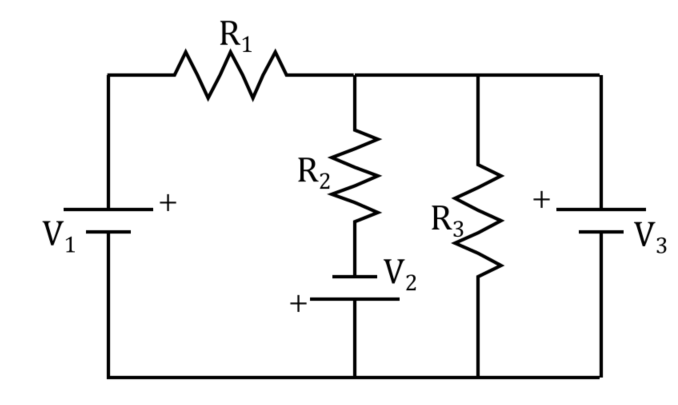# Question In the circuit below, calculate the current going through the 460 ΩΩ resistor (give the absolute value). R1=200 ΩΩ, R2=460 ΩΩ, R3=200 ΩΩ, V1=18 V, V2=20 V, V3=18 V. ____ A. Ri R2 + + R3 V1 V3 V2 + +In the circuit below, calculate the current going through the 460 ΩΩ resistor (give the absolute value).

R1=200 ΩΩ, R2=460 ΩΩ, R3=200 ΩΩ, V1=18 V, V2=20 V, V3=18 V.

____ A.Transcribed Image Text: Ri R2 + + R3 V1 V3 V2 + +
More
Transcribed Image Text: Ri R2 + + R3 V1 V3 V2 + +